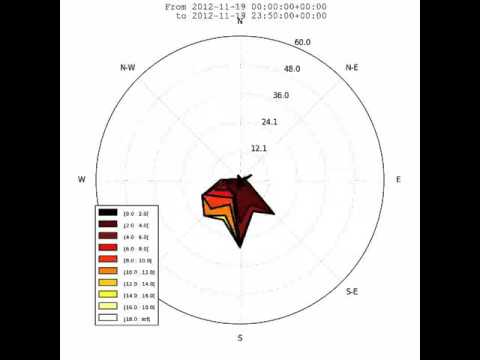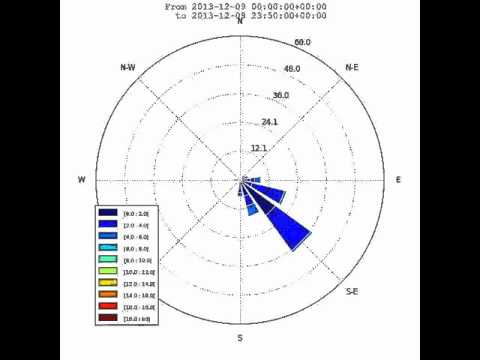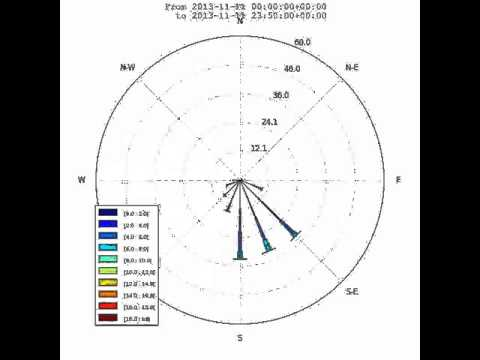# Notebook example¶

An IPython (Jupyter) notebook showing this package usage is available at:

# Script example¶

This example use randoms values for wind speed and direction(ws and wd variables). In situation, these variables are loaded with reals values (1-D array), from a database or directly from a text file (see the “load” facility from the matplotlib.pylab interface for that).

```from windrose import WindroseAxes
from matplotlib import pyplot as plt
import matplotlib.cm as cm
import numpy as np

# Create wind speed and direction variables

ws = np.random.random(500) * 6
wd = np.random.random(500) * 360
```

## A stacked histogram with normed (displayed in percent) results¶

```ax = WindroseAxes.from_ax()
ax.bar(wd, ws, normed=True, opening=0.8, edgecolor='white')
ax.set_legend()
```## Another stacked histogram representation, not normed, with bins limits¶

```ax = WindroseAxes.from_ax()
ax.box(wd, ws, bins=np.arange(0, 8, 1))
ax.set_legend()
```## A windrose in filled representation, with a controlled colormap¶

```ax = WindroseAxes.from_ax()
ax.contourf(wd, ws, bins=np.arange(0, 8, 1), cmap=cm.hot)
ax.set_legend()
```## Same as above, but with contours over each filled region…¶

```ax = WindroseAxes.from_ax()
ax.contourf(wd, ws, bins=np.arange(0, 8, 1), cmap=cm.hot)
ax.contour(wd, ws, bins=np.arange(0, 8, 1), colors='black')
ax.set_legend()
```## …or without filled regions¶

```ax = WindroseAxes.from_ax()
ax.contour(wd, ws, bins=np.arange(0, 8, 1), cmap=cm.hot, lw=3)
ax.set_legend()
```After that, you can have a look at the computed values used to plot the windrose with the `ax._info` dictionary :

• `ax._info['bins']` : list of bins (limits) used for wind speeds. If not set in the call, bins will be set to 6 parts between wind speed min and max.
• `ax._info['dir']` : list of directions “boundaries” used to compute the distribution by wind direction sector. This can be set by the nsector parameter (see below).
• `ax._info['table']` : the resulting table of the computation. It’s a 2D histogram, where each line represents a wind speed class, and each column represents a wind direction class.

So, to know the frequency of each wind direction, for all wind speeds, do:

```ax.bar(wd, ws, normed=True, nsector=16)
table = ax._info['table']
wd_freq = np.sum(table, axis=0)
```

and to have a graphical representation of this result :

```direction = ax._info['dir']
wd_freq = np.sum(table, axis=0)
plt.bar(np.arange(16), wd_freq, align='center')
xlabels = ('N','','N-E','','E','','S-E','','S','','S-O','','O','','N-O','')
xticks=arange(16)
gca().set_xticks(xticks)
draw()
gca().set_xticklabels(xlabels)
draw()
```In addition of all the standard pyplot parameters, you can pass special parameters to control the windrose production. For the stacked histogram windrose, calling help(ax.bar) will give : `bar(self, direction, var, **kwargs)` method of `windrose.WindroseAxes` instance Plot a windrose in bar mode. For each var bins and for each sector, a colored bar will be draw on the axes.

Mandatory:

• `direction` : 1D array - directions the wind blows from, North centred
• `var` : 1D array - values of the variable to compute. Typically the wind speeds

Optional:

• `nsector` : integer - number of sectors used to compute the windrose table. If not set, nsectors=16, then each sector will be 360/16=22.5°, and the resulting computed table will be aligned with the cardinals points.
• `bins` : 1D array or integer - number of bins, or a sequence of bins variable. If not set, bins=6 between min(var) and max(var).
• `blowto` : bool. If True, the windrose will be pi rotated, to show where the wind blow to (useful for pollutant rose).
• `colors` : string or tuple - one string color (`'k'` or `'black'`), in this case all bins will be plotted in this color; a tuple of matplotlib color args (string, float, rgb, etc), different levels will be plotted in different colors in the order specified.
• `cmap` : a cm Colormap instance from `matplotlib.cm`. - if `cmap == None` and `colors == None`, a default Colormap is used.
• `edgecolor` : string - The string color each edge bar will be plotted. Default : no edgecolor
• `opening` : float - between 0.0 and 1.0, to control the space between each sector (1.0 for no space)
• `mean_values` : Bool - specify wind speed statistics with direction=specific mean wind speeds. If this flag is specified, var is expected to be an array of mean wind speeds corresponding to each entry in `direction`. These are used to generate a distribution of wind speeds assuming the distribution is Weibull with shape factor = 2.
• `weibull_factors` : Bool - specify wind speed statistics with direction=specific weibull scale and shape factors. If this flag is specified, var is expected to be of the form [[7,2], …., [7.5,1.9]] where var[i] is the weibull scale factor and var[i] is the shape factor

## probability density function (pdf) and fitting Weibull distribution¶

A probability density function can be plot using:

```from windrose import WindAxes
ax = WindAxes.from_ax()
bins = np.arange(0, 6 + 1, 0.5)
bins = bins[1:]
ax, params = ax.pdf(ws, bins=bins)
```Optimal parameters of Weibull distribution can be displayed using

```print(params)
(1, 1.7042156870194352, 0, 7.0907180300605459)
```

## Overlay of a map¶

This example illustrate how to set an windrose axe on top of any other axes. Specifically, overlaying a map is often useful. It rely on matplotlib toolbox inset_axes utilities.

```import numpy as np
import matplotlib.pyplot as plt
from mpl_toolkits.axes_grid.inset_locator import inset_axes
import cartopy.crs as ccrs
import cartopy.io.img_tiles as cimgt

import windrose

ws = np.random.random(500) * 6
wd = np.random.random(500) * 360

minlon, maxlon, minlat, maxlat = (6.5, 7.0, 45.85, 46.05)

proj = ccrs.PlateCarree()
fig = plt.figure(figsize=(12, 6))
# Draw main ax on top of which we will add windroses
main_ax = fig.add_subplot(1, 1, 1, projection=proj)
main_ax.set_extent([minlon, maxlon, minlat, maxlat], crs=proj)
main_ax.gridlines(draw_labels=True)
main_ax.coastlines()

request = cimgt.OSM()

# Coordinates of the station we were measuring windspeed
cham_lon, cham_lat = (6.8599, 45.9259)
passy_lon, passy_lat = (6.7, 45.9159)

# Inset axe it with a fixed size
wrax_cham = inset_axes(main_ax,
width=1,                             # size in inches
height=1,                            # size in inches
loc='center',                        # center bbox at given position
bbox_to_anchor=(cham_lon, cham_lat), # position of the axe
bbox_transform=main_ax.transData,    # use data coordinate (not axe coordinate)
axes_class=windrose.WindroseAxes,    # specify the class of the axe
)

# Inset axe with size relative to main axe
height_deg = 0.1
wrax_passy = inset_axes(main_ax,
width="100%",                        # size in % of bbox
height="100%",                       # size in % of bbox
#loc='center',  # don't know why, but this doesn't work.
# specify the center lon and lat of the plot, and size in degree
bbox_to_anchor=(passy_lon-height_deg/2, passy_lat-height_deg/2, height_deg, height_deg),
bbox_transform=main_ax.transData,
axes_class=windrose.WindroseAxes,
)

wrax_cham.bar(wd, ws)
wrax_passy.bar(wd, ws)
for ax in [wrax_cham, wrax_passy]:
ax.tick_params(labelleft=False, labelbottom=False)
```# Functional API¶

Instead of using object oriented approach like previously shown, some “shortcut” functions have been defined: `wrbox`, `wrbar`, `wrcontour`, `wrcontourf`, `wrpdf`. See unit tests.

# Pandas support¶

windrose not only supports Numpy arrays. It also supports also Pandas DataFrame. `plot_windrose` function provides most of plotting features previously shown.

```from windrose import plot_windrose
N = 500
ws = np.random.random(N) * 6
wd = np.random.random(N) * 360
df = pd.DataFrame({'speed': ws, 'direction': wd})
plot_windrose(df, kind='contour', bins=np.arange(0.01,8,1), cmap=cm.hot, lw=3)
```

Mandatory:

• `df`: Pandas DataFrame with `DateTimeIndex` as index and at least 2 columns (`'speed'` and `'direction'`).

Optional:

• `kind` : kind of plot (might be either, `'contour'`, `'contourf'`, `'bar'`, `'box'`, `'pdf'`)
• `var_name` : name of var column name ; default value is `VAR_DEFAULT='speed'`
• `direction_name` : name of direction column name ; default value is `DIR_DEFAULT='direction'`
• `clean_flag` : cleanup data flag (remove data points with `NaN`, `var=0`) before plotting ; default value is `True`.

# Subplots¶# Video export¶

A video of plots can be exported. A playlist of videos is available at https://www.youtube.com/playlist?list=PLE9hIvV5BUzsQ4EPBDnJucgmmZ85D_b-W

See:Source code

This is just a sample for now. API for video need to be created.

Use:

```\$ python samples/example_animate.py --help
```

to display command line interface usage.